## Search Results

Results for: 'logic'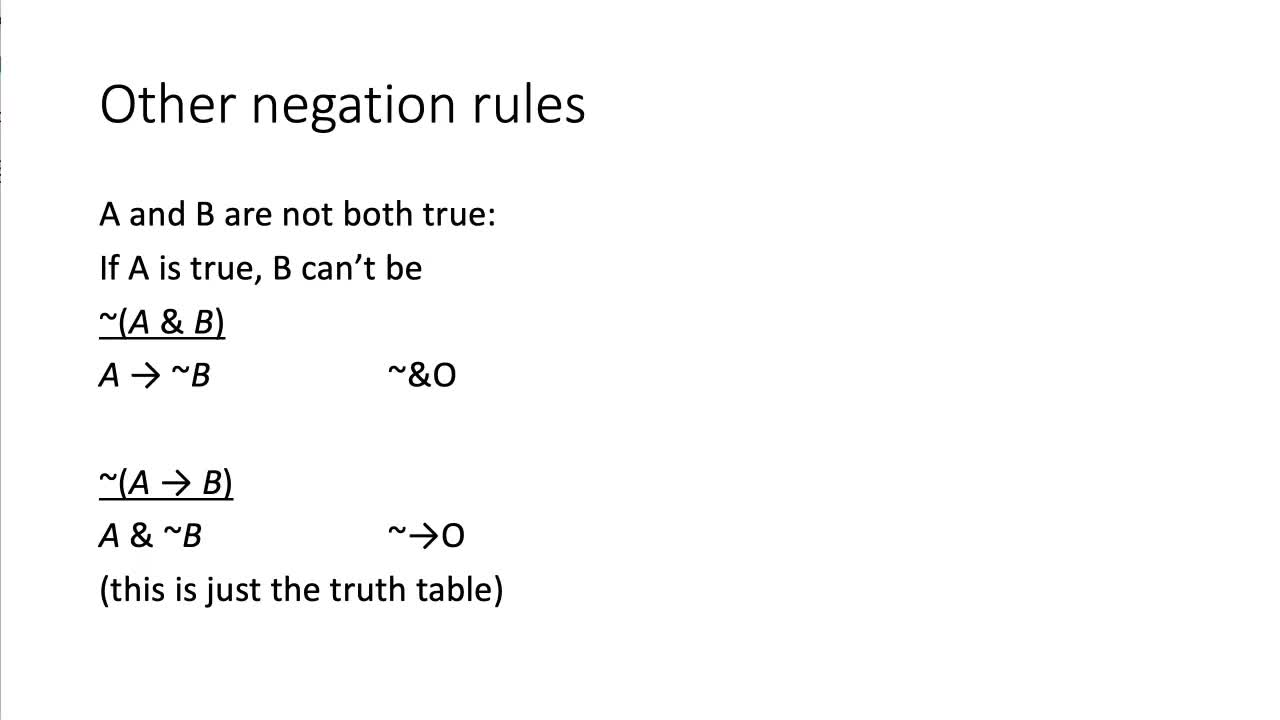7:56

By: mcweiner

A video on rules for proofs with complex negations, like ~(P & Q), ~(P v Q), and ~(P -> Q).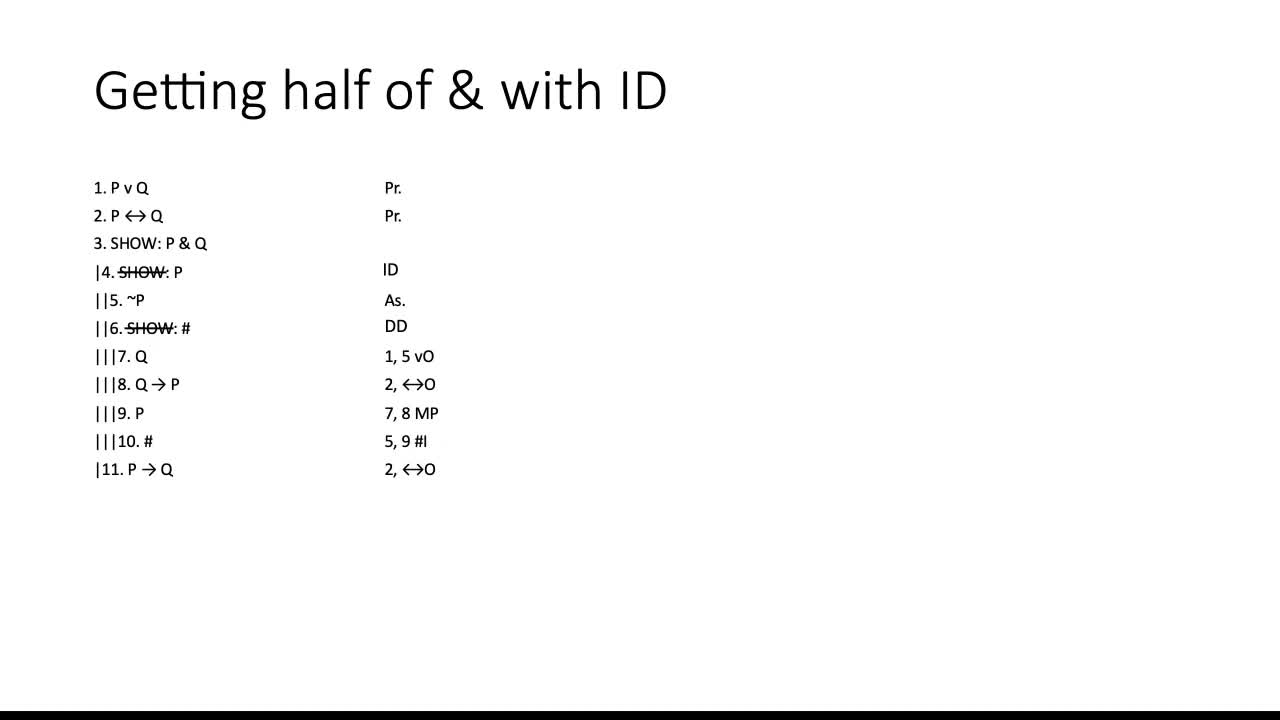7:35

By: mcweiner

A video on how to do proofs where you write down SHOW statements that don't come from specific derivation rules.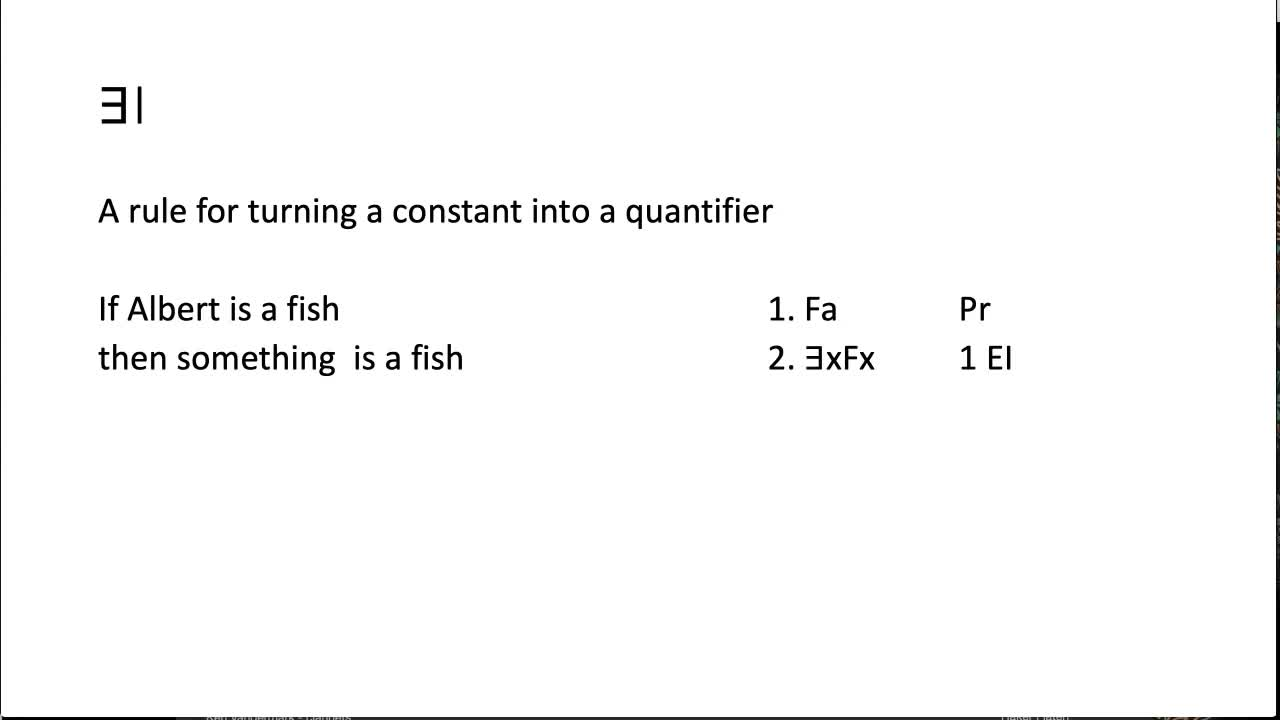7:58

By: mcweiner

A video introducing predicate proofs and the first two predicate proof rules, AO and EI.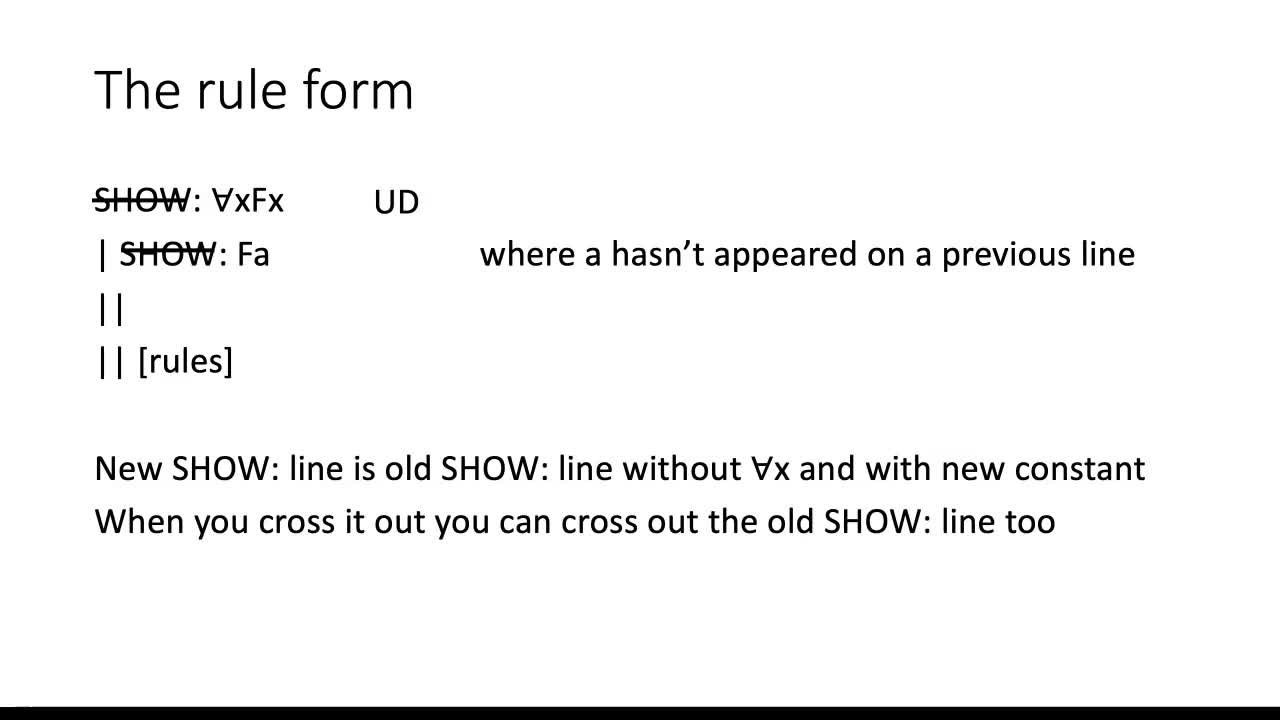5:25

By: mcweiner

A video on the Universal Derivation rule for predicate proofs, a rule for doing proofs where the SHOW line begins with Ax.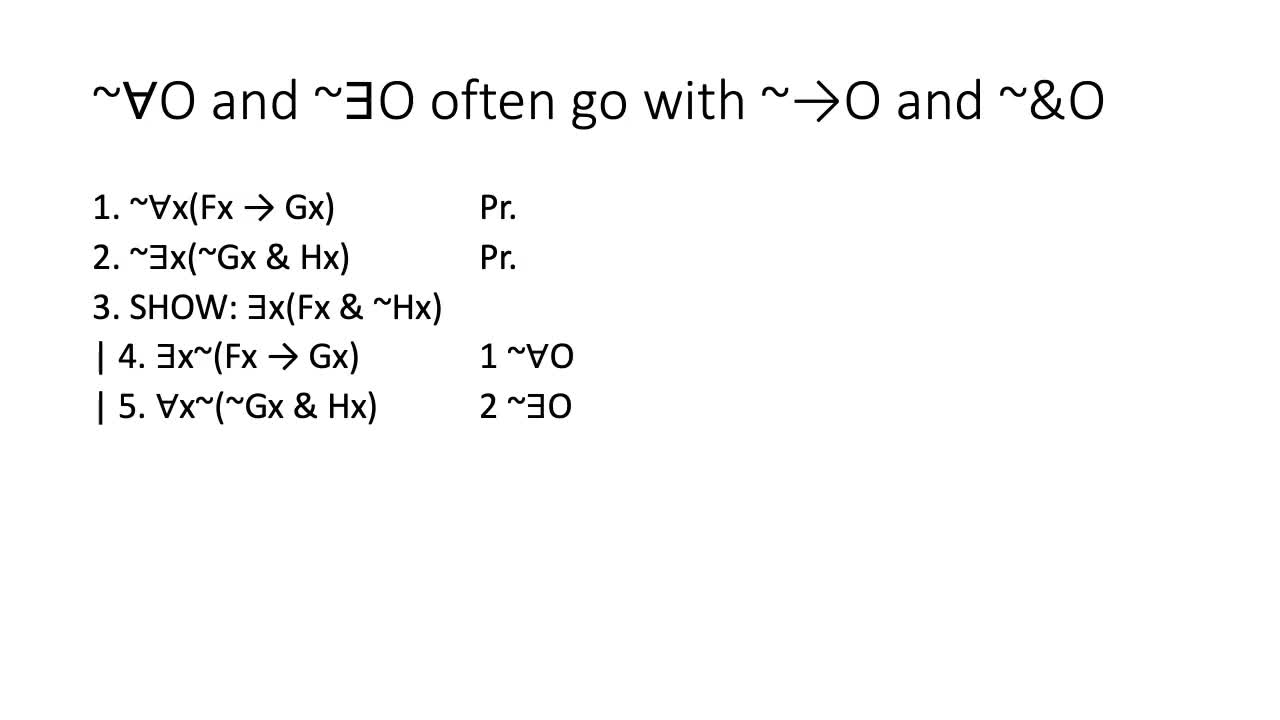9:18

By: mcweiner

A video on how to do proofs with negated quantifiers. Introduces two new rules, ~AO and ~EO, and discusses how to do proofs where you need to SHOW negated quantifiers (you have to do ID).